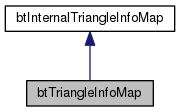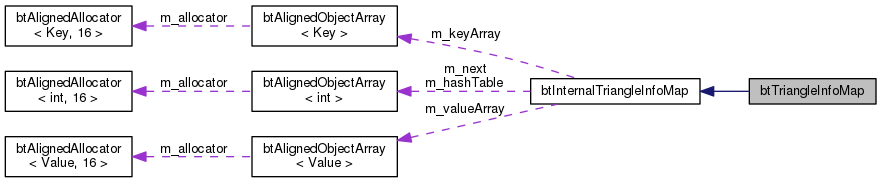Bullet Collision Detection & Physics Library
btTriangleInfoMap Struct Reference

The btTriangleInfoMap stores edge angle information for some triangles. You can compute this information yourself or using btGenerateInternalEdgeInfo. More...

`#include <btTriangleInfoMap.h>`

Inheritance diagram for btTriangleInfoMap:[legend]
Collaboration diagram for btTriangleInfoMap:[legend]

## Public Member Functions

btTriangleInfoMap ()
used to determine if a triangle is degenerate (length squared of cross product of 2 triangle edges < threshold) More...

virtual ~btTriangleInfoMap ()

virtual int calculateSerializeBufferSize () const

virtual const char * serialize (void *dataBuffer, btSerializer *serializer) const
fills the dataBuffer and returns the struct name (and 0 on failure) More...

void deSerialize (struct btTriangleInfoMapData &data)
fills the dataBuffer and returns the struct name (and 0 on failure) More...Public Member Functions inherited from btHashMap< Key, Value >
void insert (const Key &key, const Value &value)

void remove (const Key &key)

int size () const

const Value * getAtIndex (int index) const

Value * getAtIndex (int index)

Key getKeyAtIndex (int index)

const Key getKeyAtIndex (int index) const

Value * operator[] (const Key &key)

const Value * operator[] (const Key &key) const

const Value * find (const Key &key) const

Value * find (const Key &key)

int findIndex (const Key &key) const

void clear ()

## Public Attributes

btScalar m_convexEpsilon

btScalar m_planarEpsilon
used to determine if an edge or contact normal is convex, using the dot product More...

btScalar m_equalVertexThreshold
used to determine if a triangle edge is planar with zero angle More...

btScalar m_edgeDistanceThreshold
used to compute connectivity: if the distance between two vertices is smaller than m_equalVertexThreshold, they are considered to be 'shared' More...

btScalar m_maxEdgeAngleThreshold
used to determine edge contacts: if the closest distance between a contact point and an edge is smaller than this distance threshold it is considered to "hit the edge" More...

btScalar m_zeroAreaThresholdProtected Member Functions inherited from btHashMap< Key, Value >
void growTables (const Key &)Protected Attributes inherited from btHashMap< Key, Value >
btAlignedObjectArray< int > m_hashTable

btAlignedObjectArray< int > m_next

btAlignedObjectArray< Value > m_valueArray

btAlignedObjectArray< Key > m_keyArray

## Detailed Description

The btTriangleInfoMap stores edge angle information for some triangles. You can compute this information yourself or using btGenerateInternalEdgeInfo.

Definition at line 58 of file btTriangleInfoMap.h.

## Constructor & Destructor Documentation

 btTriangleInfoMap::btTriangleInfoMap ( )
inline

used to determine if a triangle is degenerate (length squared of cross product of 2 triangle edges < threshold)

Definition at line 68 of file btTriangleInfoMap.h.

 virtual btTriangleInfoMap::~btTriangleInfoMap ( )
inlinevirtual

Definition at line 77 of file btTriangleInfoMap.h.

## Member Function Documentation

 int btTriangleInfoMap::calculateSerializeBufferSize ( ) const
inlinevirtual

Definition at line 117 of file btTriangleInfoMap.h.

 void btTriangleInfoMap::deSerialize ( struct btTriangleInfoMapData & data )
inline

fills the dataBuffer and returns the struct name (and 0 on failure)

Definition at line 211 of file btTriangleInfoMap.h.

 const char * btTriangleInfoMap::serialize ( void * dataBuffer, btSerializer * serializer ) const
inlinevirtual

fills the dataBuffer and returns the struct name (and 0 on failure)

Definition at line 123 of file btTriangleInfoMap.h.

## Member Data Documentation

 btScalar btTriangleInfoMap::m_convexEpsilon

Definition at line 60 of file btTriangleInfoMap.h.

 btScalar btTriangleInfoMap::m_edgeDistanceThreshold

used to compute connectivity: if the distance between two vertices is smaller than m_equalVertexThreshold, they are considered to be 'shared'

Definition at line 63 of file btTriangleInfoMap.h.

 btScalar btTriangleInfoMap::m_equalVertexThreshold

used to determine if a triangle edge is planar with zero angle

Definition at line 62 of file btTriangleInfoMap.h.

 btScalar btTriangleInfoMap::m_maxEdgeAngleThreshold

used to determine edge contacts: if the closest distance between a contact point and an edge is smaller than this distance threshold it is considered to "hit the edge"

Definition at line 64 of file btTriangleInfoMap.h.

 btScalar btTriangleInfoMap::m_planarEpsilon

used to determine if an edge or contact normal is convex, using the dot product

Definition at line 61 of file btTriangleInfoMap.h.

 btScalar btTriangleInfoMap::m_zeroAreaThreshold

Definition at line 65 of file btTriangleInfoMap.h.

The documentation for this struct was generated from the following file: